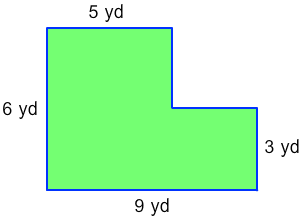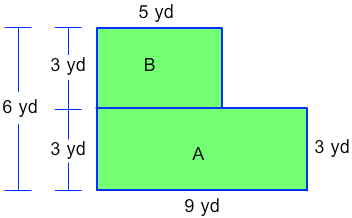# Area of Complex Figures (With All Right Angles)

Rate 0 stars
Common Core
Quiz size:
Message preview:
Someone you know has shared Area of Complex Figures (With All Right Angles) quiz with you:

To play this quiz, click on the link below:

https://www.turtlediary.com/quiz/area-of-complex-figures-with-all-right-angles.html

Hope you have a good experience with this site and recommend to your friends too.

Login to rate activities and track progress.
Login to rate activities and track progress.

Consider this figure:Let's find the area of this figure.

To find the area, we need to divide the figure into smaller figures.The given figure can be divided into 2 rectangles A and B.

Length of rectangle A = 9 yards

Width of rectangle A = 3 yards

We know that:

Area of a rectangle = length × width

Using formula,

9 x 3 = 27

The area of rectangle A is 27 square yards.

Now, look at rectangle B.

Length of rectangle B = 5 yards

Width of rectangle B = 3 yards

Using formula,

5 x 3 = 15

The area of rectangle B is 15 square yards.

Now add the areas of both the rectangles:

27 + 15 = 42

So, the total area of the given figure is 42 square yards.

ds

A B C D E F G H I J K L M N O P Q R S T U V W X Y Z

### Help

##### Remember :

The smallest number is the one that comes first while counting.

##### Solution :

To arrange the given numbers in order from smallest to greatest, find the smallest number among all the given numbers.

21,27,23

21 is the smallest number.